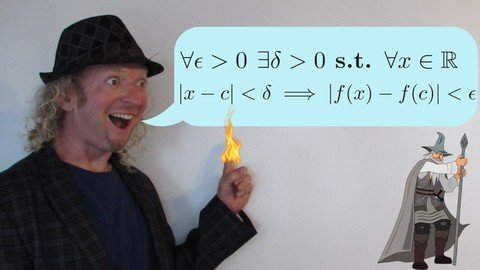# Advanced Calculus Real Analysis with the Math SorcererGenre: eLearning | MP4 | Video: h264, 1280x720 | Audio: aac, 44100 Hz
Language: English | SRT | Size: 3.71 GB | Duration: 7h 18m
What you'll learn

### Advanced Calculus Real Analysis with the Math SorcererHow to Write Proofs in Advanced Calculus
How to Prove the Triangle, Reverse Triangle, and Bernoulli's Inequality
How to Prove Various Important Results Regarding Sequences
How to Prove the Squeeze Theorem
How to Prove a Sequence Converges
How to Prove a Sequence Diverges
How to Prove Results Regarding Cauchy Sequences
How to Prove a Sequence is Cauchy
How to Write a Delta Epsilon Proof
How to Prove a Function is Continuous
How to Prove a Function is Uniformly Continuous
How to Prove Pointwise Convergence
How to Prove Uniform Convergence
How to Prove a Function is Differentiable
How to Prove a Function is Not Differentiable
Understand the Convergence of Infinite Series
How to Use Dirichlet's Test
How to Find a Pointwise Limit
How to Prove Various Key Results in Advanced Calculus
Requirements
Calculus 1, 2, 3, Differential equations + some proof writing experience is the typical recommendation for this material
A strong desire to learn higher level mathematics
Description
This is a University Level course on Selected Topics in Advanced Calculus/Real Analysis with a major focus on WRITING PROOFS:)

Note: Advanced Calculus(aka Real Analysis) is typically considered the HARDEST course a mathematics major will take.

This course is a step above a general mathematics course. Students should have familiarity with writing proofs and mathematical notation.

Basically just,

1) Watch the videos, and try to follow along with a pencil and paper, take notes!

2) Try to learn to write the proofs as I do. If you understand the proofs then you have learned a great deal. If you can write the proofs on your own then you have really graduated to the next level.

3) Repeat!

If you finish even 50% of this course you will know A LOT of Advanced Calculus and more importantly your level of mathematical maturity will go up tremendously!

Advanced Calculus is a beautiful yet notoriously difficult subject to learn and teach. I hope you enjoy watching these videos and working through these problems as much as I have:)

Who this course is for:
Math Majors
Anyone with a strong desire to learn higher level mathematics

or### Advanced Calculus Real Analysis with the Math Sorcerersupnatural ||Someone else's computer Forgot your password? Create an account

### Friends